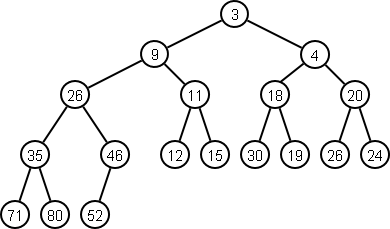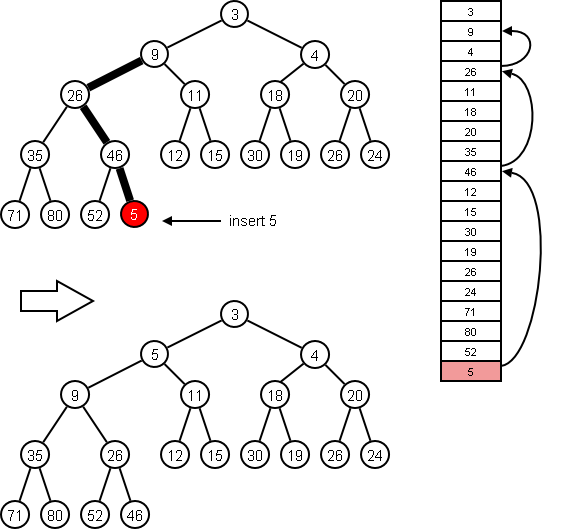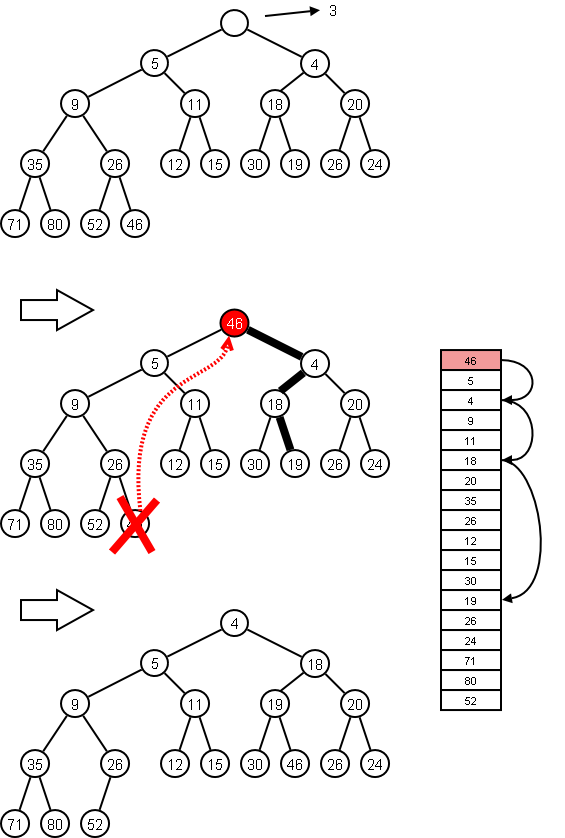# Priority Queues

Priority queues are among the most useful of all collections.

## The Basics

A priority queue is a collection in which items can be added at any time, but the only item that can be removed is the one with the highest priority.

Operations

• add(x): add item $x$
• remove: remove the highest priority item
• peek: return the highest priority item (without removing it)
• size: return the number of items in the priority queue
• isEmpty: return whether the priority queue has no items

## Examples

• Patients in an emergency room
• Operating system scheduler
• Routing
• A* search
• Simulation
• Many more examples

## Representations

Unsorted Array

• add is $\Theta(1)$ — just add the item at the end.
• peek is $\Theta(n)$ — must do linear scan to find.
• remove is $\Theta(n)$ — must find the element and compress the array.

• add is $\Theta(1)$ — just add the item at the end.
• peek is $\Theta(n)$ — must do linear scan to find.
• remove is $\Theta(n)$ — because the item must be found first.

Sorted Array

• add is $\Theta(n)$ — you can find where to insert in log time, but you have to make room in the array, which is linear.
• peek is $\Theta(1)$ — the value is at the end of the array.
• remove is $\Theta(1)$ — we can just whack the item off the end.

• add is $\Theta(n)$ — you have to step through the chain to find the place to insert.
• peek is $\Theta(1)$ — the value is at the end of the array.
• remove is $\Theta(1)$ — we can just whack the item off the end.

Heap

• add is $\Theta(\log n)$
• peek is $\Theta(1)$
• remove is $\Theta(\log n)$

What’s a heap, anyway?

### Heaps

A heap is a complete binary tree in which the value of a node is less than all the values in its subtrees. By convention, the smallest element is the one with the highest priority.#### Peek

Therefore, the smallest element, the one with the highest priority, is always on top, so peek() is $\Theta(1)$.

To insert, add the new item to the last slot in the array and sift it up.Since the tree is complete, it has minimum height, and the worst case number of moves up is logarithmic in the heap size. So cool.

#### Remove

To remove, return the value at the top, replace the top node with the value of the last slot, destroy the last slot, and sift down.It should be pretty easy to prove that deletion is done in logarithmic time.

#### Heapify

A cool operation you’ll sometimes see is called heapify — it turns a random array into a heap:

for (int i = a.length/2; i >= 0; i--) {
siftdown(i);
}

Exercise: Show this in action on the following array [33 41 7 15 55 86 28 22 94 9 11 10 8 99 16 27 51 83 2]

#### More on Heaps

By the way...

• The heap described above is really a binary heap.
• A binary heap is just a d-ary heap with $d=2$.
• You might be interested in Fibonacci heaps, Pairing heaps, Skew heaps, Soft heaps, Binomial heaps, Leftist heaps, and Beaps.
Exercise: Write a survey article on the different kinds of heaps.

## Priority Queues in Java

There’s already a PriorityQueue class in the Java Core API. It implements the Queue interface, and has the following characteristics:

• It is implemented with a heap.
• It uses the element type’s natural ordering or a comparator passed to the constructor.
• The highest priority element is the smallest element.
• add(), offer(), poll(), and remove() run in $\Theta(\log n)$ time.
• peek(), element(), and size() run in $\Theta(1)$ time.
• The convenience methods remove(Object) and contains(Object) run in Θ(n) time.

There’s also the PriorityBlockingQueue class.

### Implementation From Scratch

We’re going to write a priority 1ueue class from scratch because:

• This is the only way for you to really learn how priority queues work.
• You need coding practice.
• You need to know how to code things up when you find yourself in a very restrictive environment that doesn’t have a collections library.
We’ll develop this during class

### Unit Testing

Here are some of the things we need to test:

• A queue is empty on construction
• A queue has size 0 on construction
• After $n$ enqueues to an empty queue, $n > 0$, the queue is not empty and its size is $n$
• If the size is $n$, then after $n$ dequeues, the queue is empty and has size 0
• If one enqueues the values 1 through 50, in some random order, into an empty queue, then if 50 dequeues are done the values dequeued are 1 through 50, respectively
• Dequeueing from an empty queue throws a NoSuchElementException
• Peeking into an empty queue throws a NoSuchElementException

Here is a rough unit test:

We’ll develop this during class

## Priority Queues in Ruby

Here’s a binary heap-based, non-thread-safe, non-blocking, implementation in Ruby:

priorityqueue.rb
class PriorityQueue
def initialize
@heap = []
end

@heap << x
sift_up(@heap.length - 1)
self
end

def peek
@heap
end

def remove!()
raise RuntimeError, "Empty Queue" if @heap.length == 0
if @heap.length == 1
@heap = []
else
@heap = @heap.pop
sift_down(0)
end
self
end

def to_s
@heap.to_s
end

private

# Sift up the element at index i
def sift_up(i)
parent = (i - 1) / 2
if parent >= 0 and @heap[parent] > @heap[i]
@heap[parent], @heap[i] = @heap[i], @heap[parent]
sift_up(parent)
end
end

# Sift down the element at index i
def sift_down(i)
child = (i * 2) + 1
return if child >= @heap.length
child += 1 if child + 1 < @heap.length and @heap[child] > @heap[child+1]
if @heap[i] > @heap[child]
@heap[child], @heap[i] = @heap[i], @heap[child]
sift_down(child)
end
end
end

Exercise: Add methods heapify, empty?, and size.

And here’s a starter unit test (it only tests that add and remove work; it does not test any low-level details of sifting.

priorityqueuetest.rb
require 'minitest/autorun'
require './priorityqueue.rb'

class PriorityQueueTest < MiniTest::Test

def test_new
q = PriorityQueue.new
assert_equal(q.peek, nil)
assert_raises(RuntimeError) {q.remove!}
end

# Add 1..100 in at random, they should come out in order